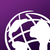# Possible BUG when using field calculator to copy value from joined table with definition query

157
0
05-03-2022 07:52 AM
Labels (1)New Contributor II

Hi,

I've run into a problem where the field calculator returns wrong values when I use it to copy the values from a joined table with an active definition query.

I used the Tabulate Intersection Tool to calculate the percentage of shape area that overlap with features in another feature class (zone field = OID of feature class 1, class field = 'Typ' of feature class 2). The class field ('Typ') contains the values VRG or VBG.

I then wanted to fill the fields 'VRG %' and 'VBG %' in feature class 1 with the respective value from the intersection table. E. g. if a feature has a 60% overlap with VRG and 25% overlap with VBG (15% not overlapping any), then the value in 'VRG %' and 'VBG %' should be 60 and 25, respectively.

I used a definition query on the intersection table to get only rows with type VRG. Then I joined the table to the feature class. The correct rows are joined. I then used the field calculator to copy the values from 'PERCENTAGE' (joined table) to 'VRG %'.

However, in many rows the calculator didn't copy the correct values but, apparently, the percentage of VBG (which were filtered out by the definition query).(I set the number format in 'VRG %' and 'VBG %' to 2 significant digits, so some rows, e.g. 18 and 23 where calculated correctly).

When filling the 'VBG %' field the error doesn't seem to occur.

The problem is similar to the one addressed here, but in my case the join works correctly and the error only occurs when using the field calculator.

Is this a known bug? Does anyone else have this problem?

I'll use a workaround for the moment, but this issue should be looked into. I'm working with ArcGIS Pro 2.9.0

Tags (3)
0 Replies